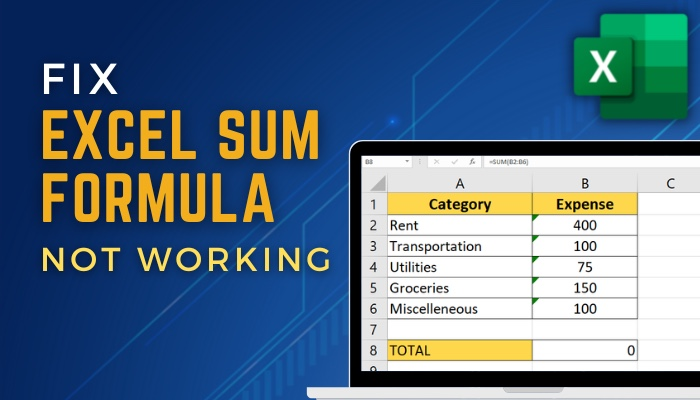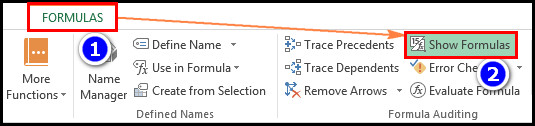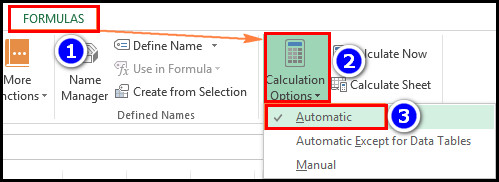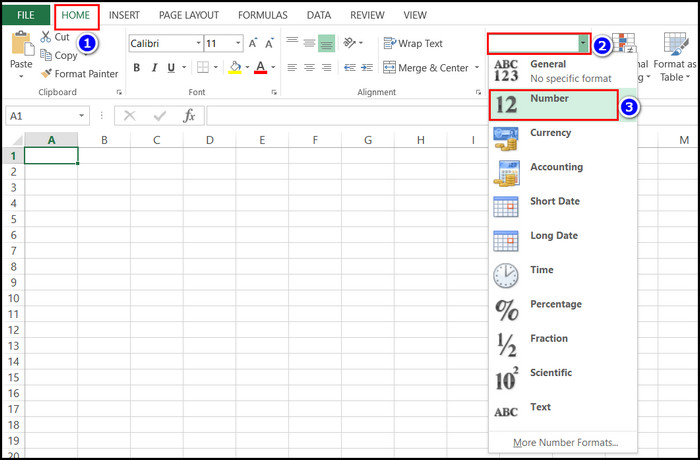# Fix Excel SUM Formula Not Working [2023 Troubleshoot Guide]Written By Steven Arends

Are you struggling to calculate your company’s budget, sales, or student test scores using Excel’s SUM formula? You rechecked the data and inputted the formula repeatedly, but nothing happened.Don’t worry; you’re not alone! The good news is you can take some simple steps to troubleshoot the issue quickly. This article will guide you through the common SUM formula issues of Excel and how to fix them.

So without further ado, let’s fix the issue and get back to working with your data.

## How to Fix Excel SUM Formula Not Working Issue

You can quickly fix issues with Excel’s SUM formula by turning the show formulas mode off and enabling the automatic recalculation option. If that doesn’t solve your issue, check for any extra space, double quotes, or separators, and remove them.

In this portion, I will show you a step-by-step guide for all the methods available to solve SUM formula working issues in Microsoft Excel. Let’s see how to do it.

Here are the fixes for the SUM formula not working issue in MS Excel:

### 1. Turn Off Show Formulas Mode

When you inadvertently activate the Show Formulas mode in a worksheet, the SUM of Microsoft Excel won’t work. You just need to disable the option to fix this issue.

Follow the steps below to disable show formulas mode:

• Open the Excel file.
• Navigate to the Formulas tab > Formula Auditing group.
• Click the Show Formulas button. Or, press Ctrl + ` keys on your keyboard.After completing the steps, the SUM formula will start working again. You should also round up the numbers on your excel sheet to get accurate results for decibel numbers.

### 2. Turn On Automatic Recalculation

When you set calculation options as manual, the results won’t update even after changing the values. And in that case, the SUM formula will display incorrect results. You need to turn on the Automatic Recalculation feature to solve the issue.

To turn on automatic recalculation, follow the steps below:

• Open the Excel file.
• Navigate to the Formulas tab > Calculation group.
• Tap on the Calculation Options and choose Automatic.You can use this method for Microsoft Excel 2010, 2013, 2016, 2019, and 2021.

However, for Excel 2007, you must go to Office icon > Excel options > Formulas > Workbook Calculation and select Automatic, then Ok.

### 3. Change Formula Formats

The SUM formula only calculates numeric values, but sometimes the cell format shows Text. When that happens, you won’t get accurate results from your excel sheet. So you need to change the cell formats.

Here are the steps to change the cell formats in Microsoft Excel:

• Select the Cells for the SUM formula.
• Go to the Number section of the Home ribbon.
• Choose the Numbers option in the box.After completing the steps, you will see the current results in the SUM formula. You can also clear the formatting of your Excel sheet to set the correct cell formats.

### 4. Remove Extra Space, Double Quotes, Separator

If you enclose values in double quotes or with extra space in Microsoft Excel, It will treat them as text strings.

So you must remove any extra space, double quotes, or separator while performing a SUM calculation.

### 5. Meet Formula Requirements

You can use up to 64 nested functions in Excel 2016, 2013, 2010, and 2007, and Excel 2003 only supports 7 nested functions. The SUM function will stop if you insert more formulas than the limit. And sometimes, Microsoft Excel can also freeze or slow for this reason.

So you need to meet the formula requirements of Microsoft Excel.

### 6. Insert Full Path to a Closed Workbook

When you write a formula as a reference from a closed Excel workbook, you must include the workbook name and the entire path to the workbook in the SUM formula.

The SUM formula will only display proper results if you insert a complete path. That’s why you need to insert the proper Path to a Closed Workbook in the SUM equation like =SUM(‘D:\Reports\[Sales.xlsx]Jan’!B2:B10).

After applying all the fixes, you won’t face any issues with the SUM function of Microsoft Excel. You should also highlight and remove duplicate values in Excel to get proper SUM results.

## Why Doesn’t your Excel Sum Formula Work and Return 0?

Mainly the SUM formula stops working when you insert the wrong cell reference, space, or non-numeric characters. Also, if there are some formatting issues or merged cells, separators, or double quotes in the SUM range, the formula will show incorrect results.

In this portion, I will show you some common reasons why the sum formula stops working. It will help you avoid those human mistakes.

Here are the main reasons behind Sum Formula not working in Microsoft Excel:

### Incorrect Cell References

You will get no or wrong results If the cell references in the SUM formula are incorrect. So recheck the cell reference while inserting the SUM formula.

### Empty Cells Within Range

Excel’s SUM formula doesn’t include empty cells while calculating. Including blank cells in the equation can lead to unexpected results.

### Non-Numeric Characters In the Cell

The SUM formula only calculates numeric values. So any non-numeric characters or text in the cells will cause an error in results.

### Formatting Issues with the Cells

Excel formulas can only recognize the cell values properly if you format them as numbers. So you should ensure the data formats as numbers.

### Locked Or Merged Cells

Locking a cell in Microsoft Excel is an excellent way to protect your data & formula. But the SUM formula can only calculate the cells that are not merged or locked.

So if you have any merged cells within the sum range, unmerge those cells from the excel sheet or exclude them.

These are the main reasons behind the SUM formula not working issue in Microsoft Excel. So you need to be careful while inserting the data and formulas. Then, you can avoid most of the errors of the SUM formula.

## FAQs

How many arguments can the SUM formula take?

The SUM formula can take up to 255 arguments in Microsoft Excel.

Can you do a Sumif with 3 criteria in MS Excel?

No, SUMIF can evaluate just one condition simultaneously, but you can use the SUMIFS to check multiple criteria.

Can you have two sum ranges in Sumifs?

Yes, you can insert two or more SUMIF or use a combination of SUM and SUMIF functions to calculate two ranges.

## Conclusion

Microsoft Excel’s SUM formula is a powerful tool for calculating the total of a range of cells. But, sometimes, the formula won’t give the expected results for script bugs or wrong data input.

When that happens, you should turn off show formulas mode, enable automatic recalculation, and remove any extra space or double quotes to solve your issue.

After applying the solutions, I’m sure you solved all your problems regarding the SUM formula in Microsoft Excel. Comment if you need further assistance regarding Excel. Peace out!